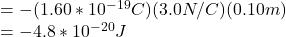Question

A proton moves 10.0 cm on a path parallel to the direction of a uniform electric field of strength 3.0 N/C. What is the change in electrical potential energy?

1.ΔPE= -4.8×10⁻²⁰J

Explanation:

Given data

Electric field of strength E=3.0 N/C

Charge of proton q=1.60×10⁻¹⁹C

Proton moves distance d=10 cm=0.10 m

To find

Change in electrical potential energy ΔPE

Solution

As we know that:

ΔPE= -qEdΔPE= -4.8×10⁻²⁰J

2.Explanation:

Given:

D = 10 cm

= 0.1 m

E = 3.0 N/C

Qp = 1.602 × 10^-19 C

U = Q × V

But,

V = E × D

= 3 × 0.1

= 0.3 V

U = 1.602 × 10-19 × 0.3

= 4.806 × 10^-20 J.